# RD Sharma Solutions for Class 8 Maths Chapter 6 - Algebraic Expressions and Identities Exercise 6.4

Students can avail free pdf of RD Sharma Solutions for Class 8 Maths Exercise 6.4 of Chapter 6 Algebraic Expressions and Identities which are provided here. In Exercise 6.4 of Chapter 6 algebraic expressions and identities, we shall discuss problems based on the multiplication of a monomial and a binomial. Our expert tutors at BYJU’S have solved the questions from the RD Sharma textbook in a step by step manner for easy understanding of the concepts, which helps students increase their speed in solving the questions quickly in their exams. The pdf of RD Sharma Solutions for class 8 chapter 6 exercise 6.4 can be downloaded easily from the links provided below.

## Download the pdf of RD Sharma Solutions For Class 8 Maths Exercise 6.4 Chapter 6 Algebraic Expressions and Identities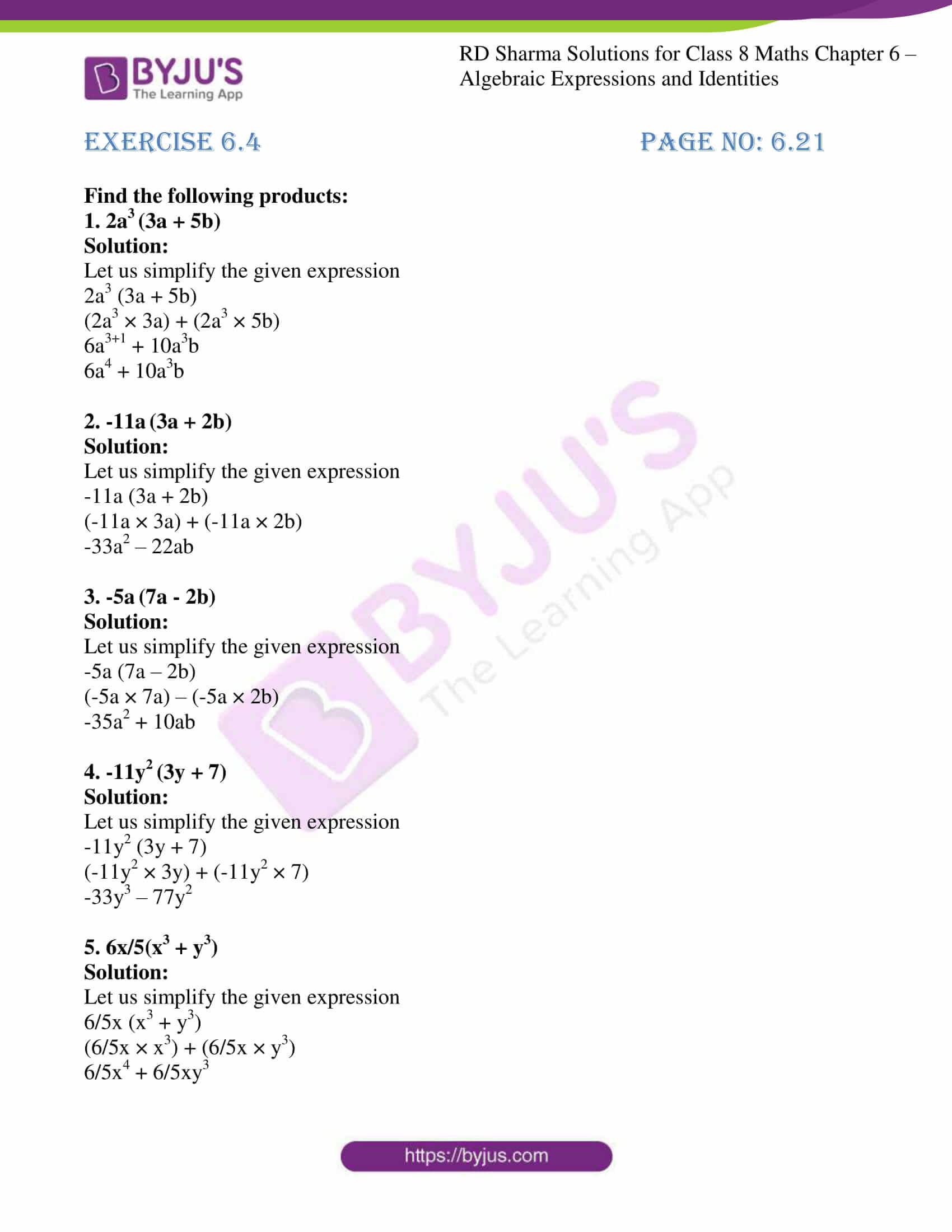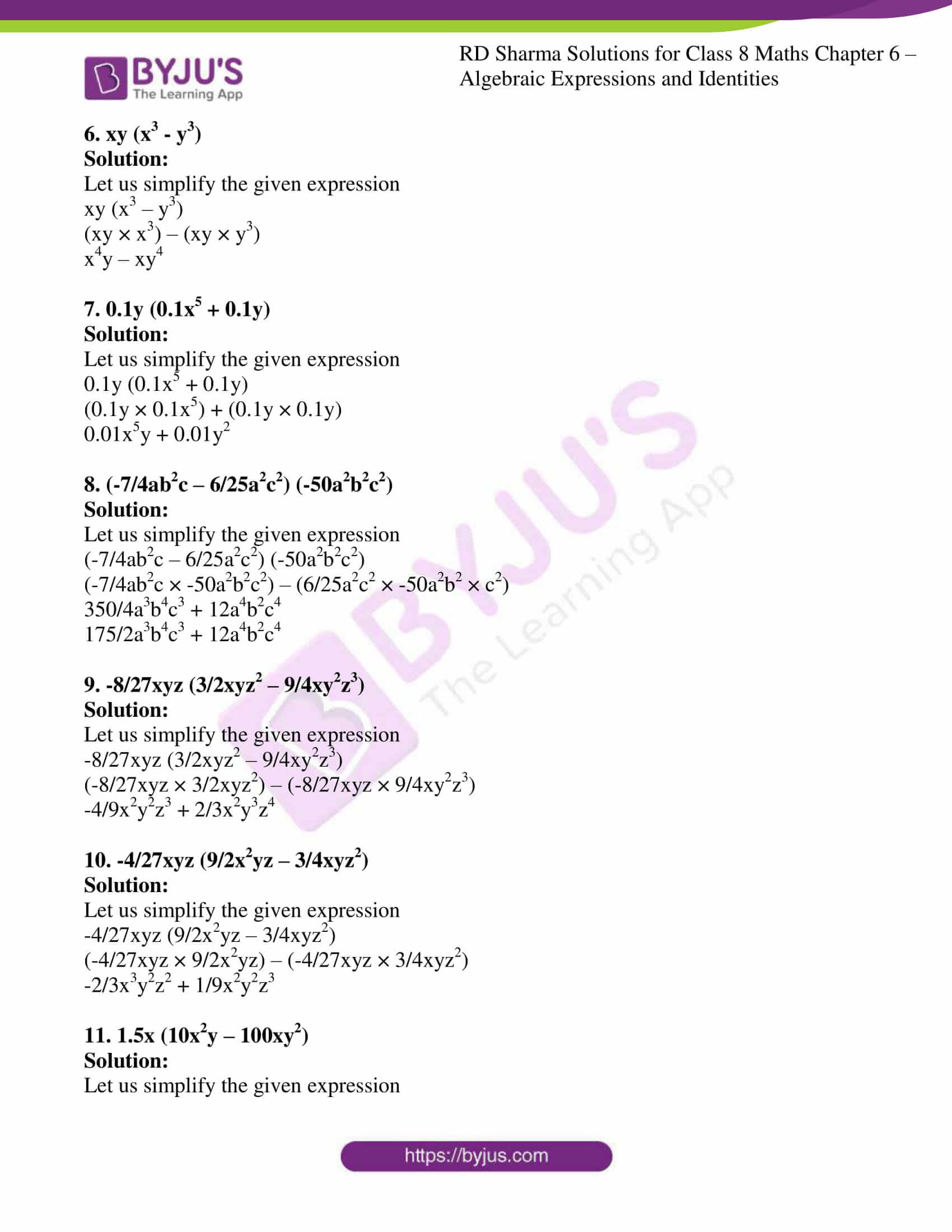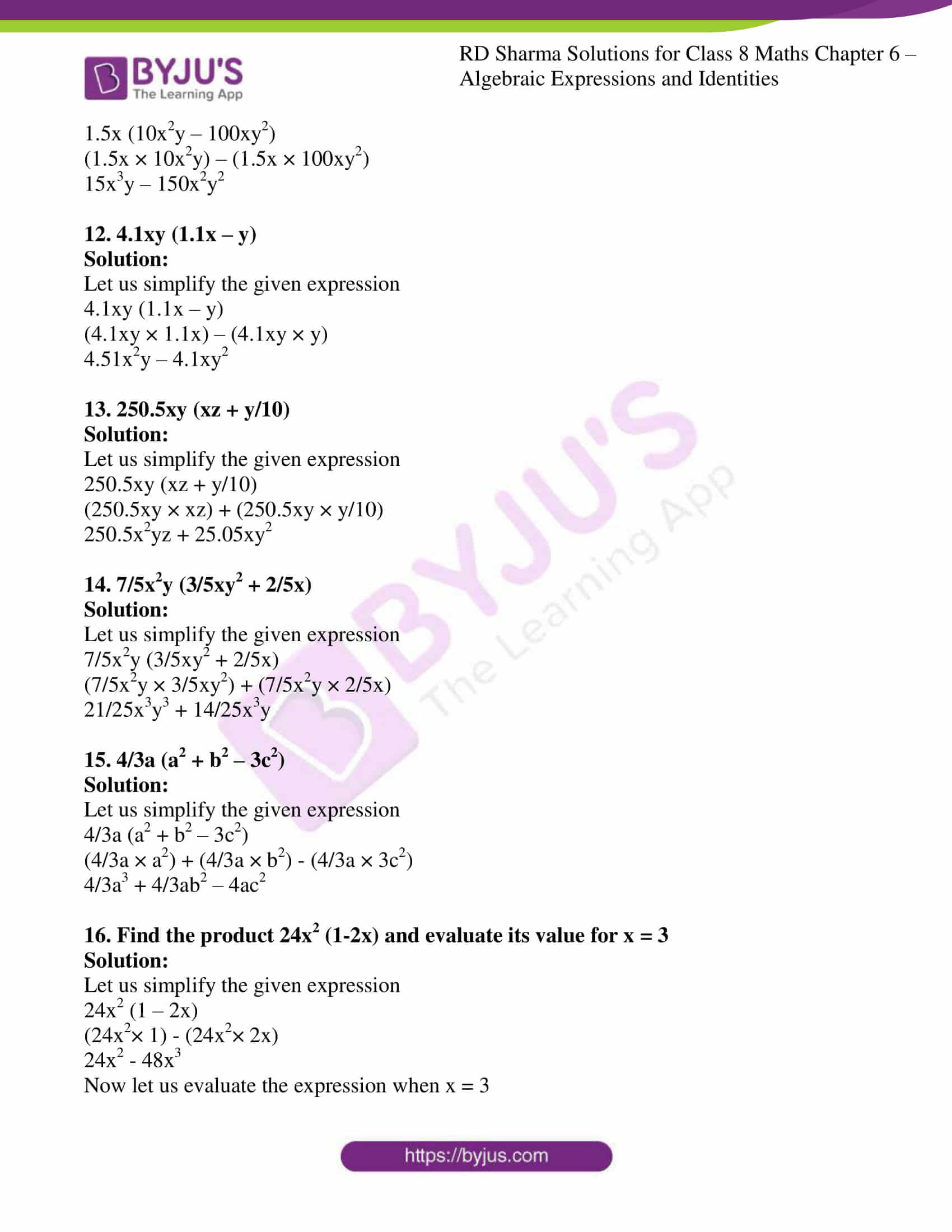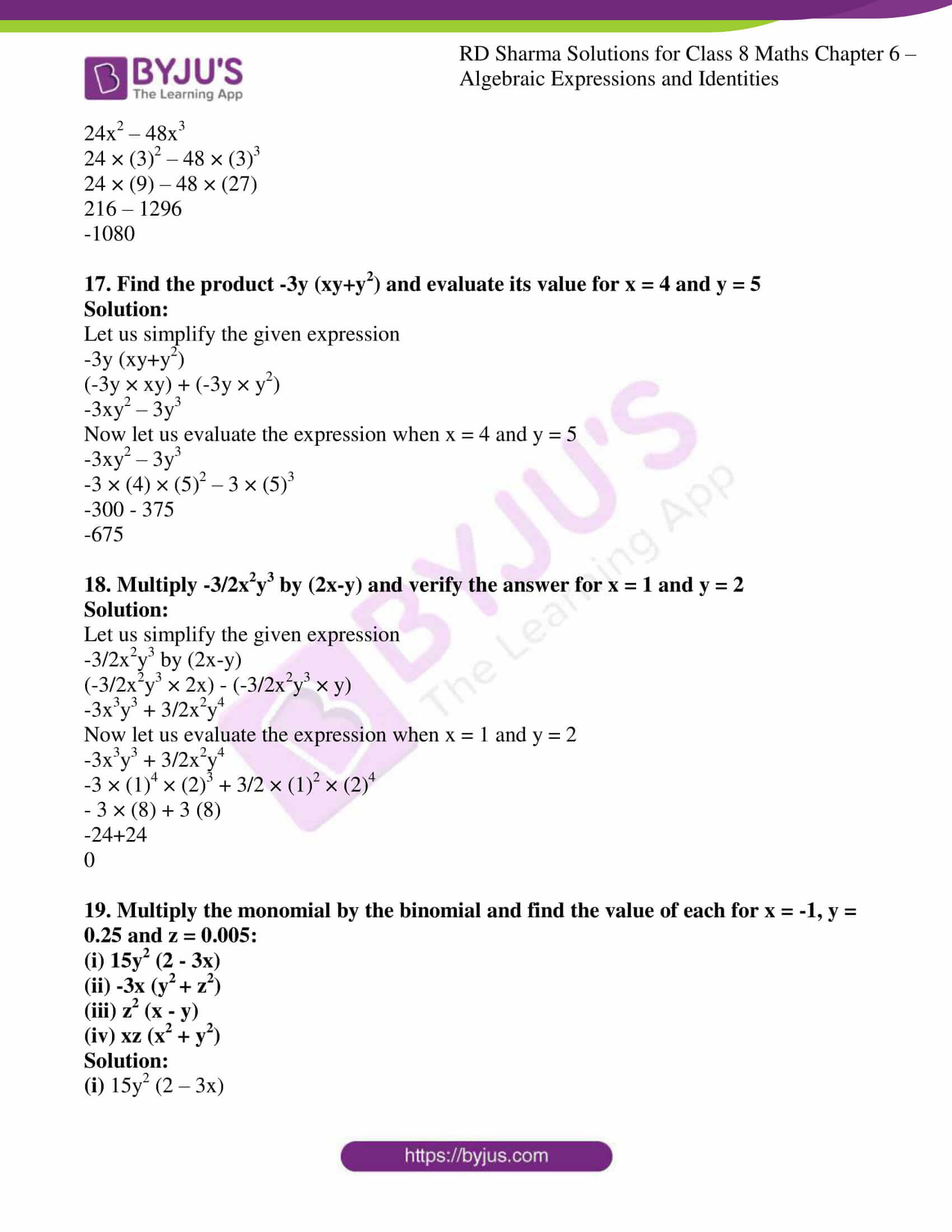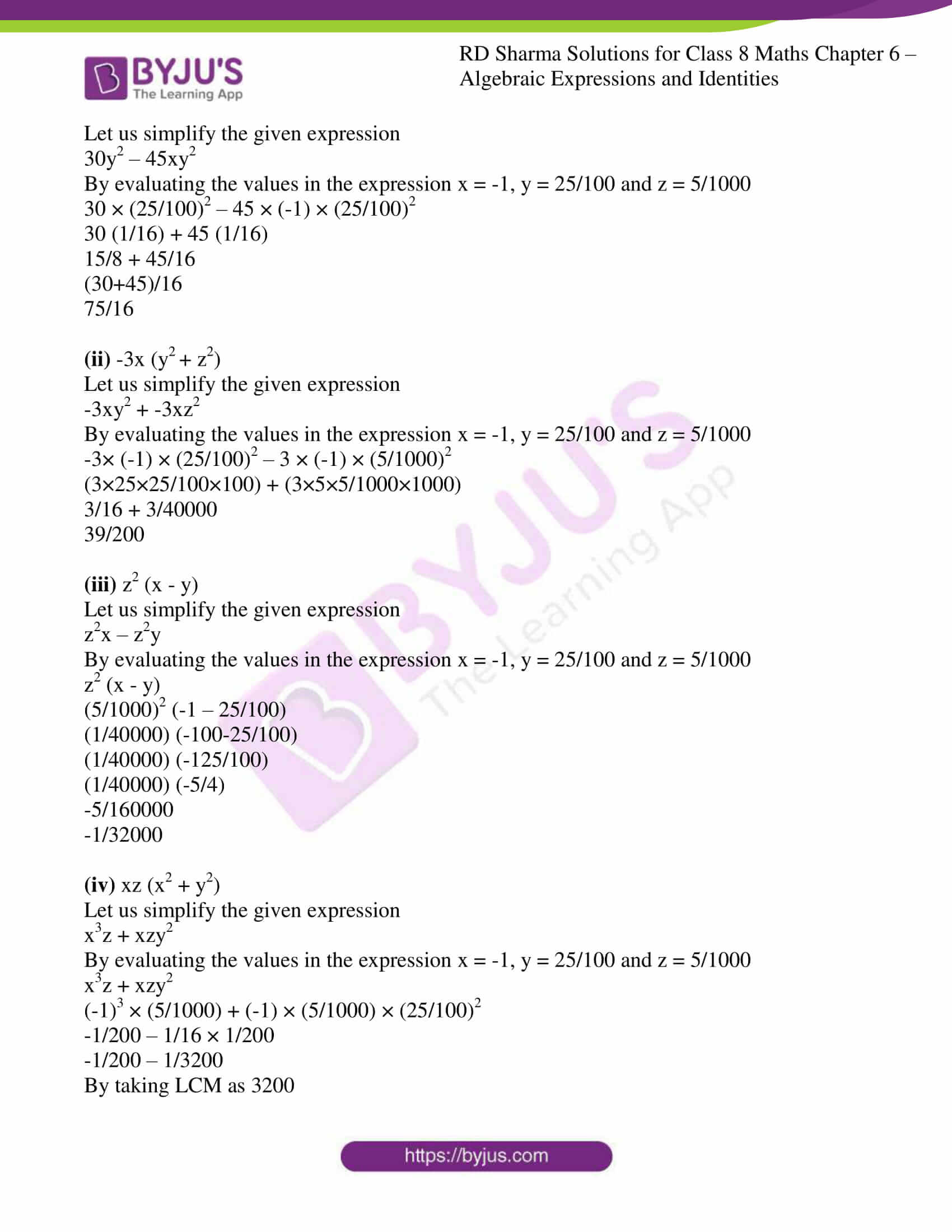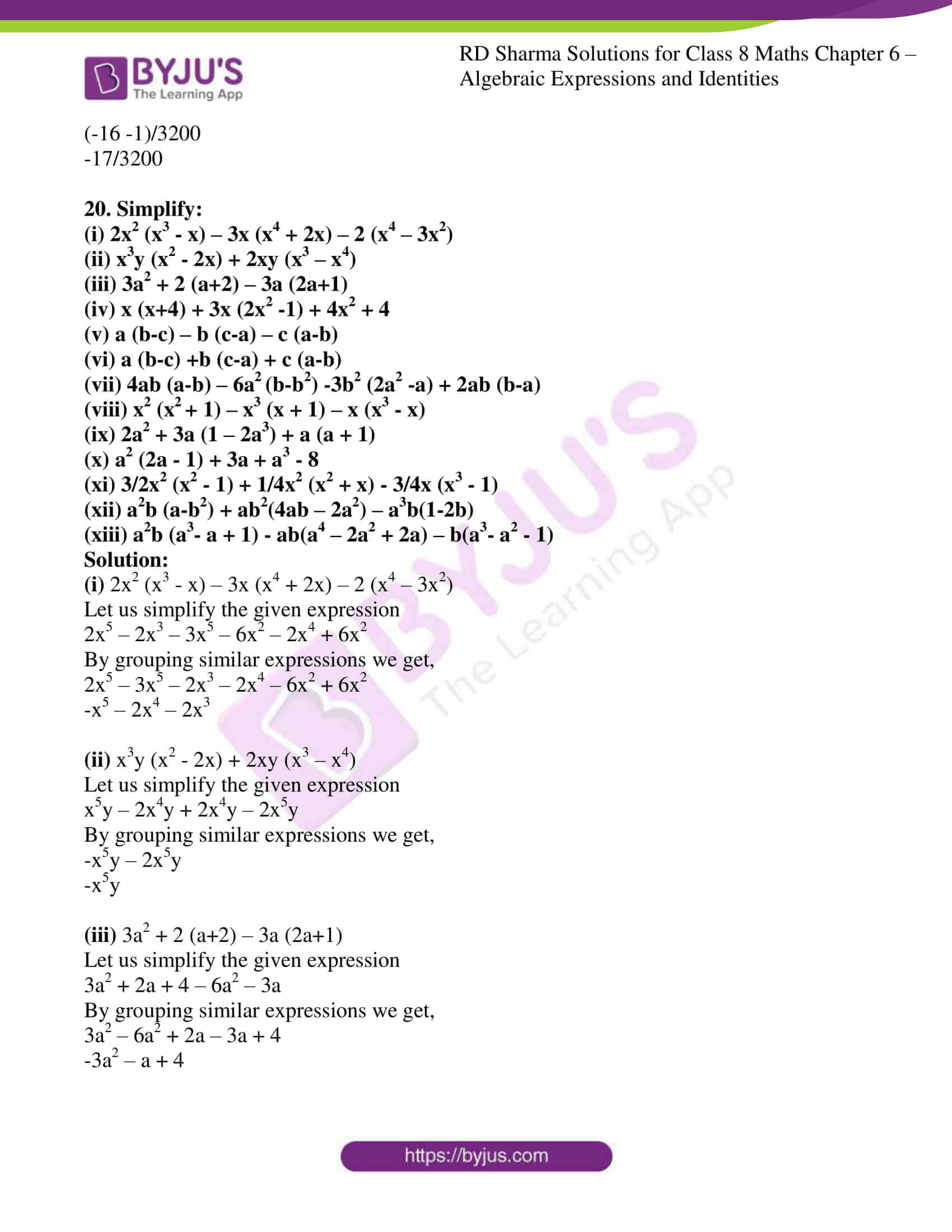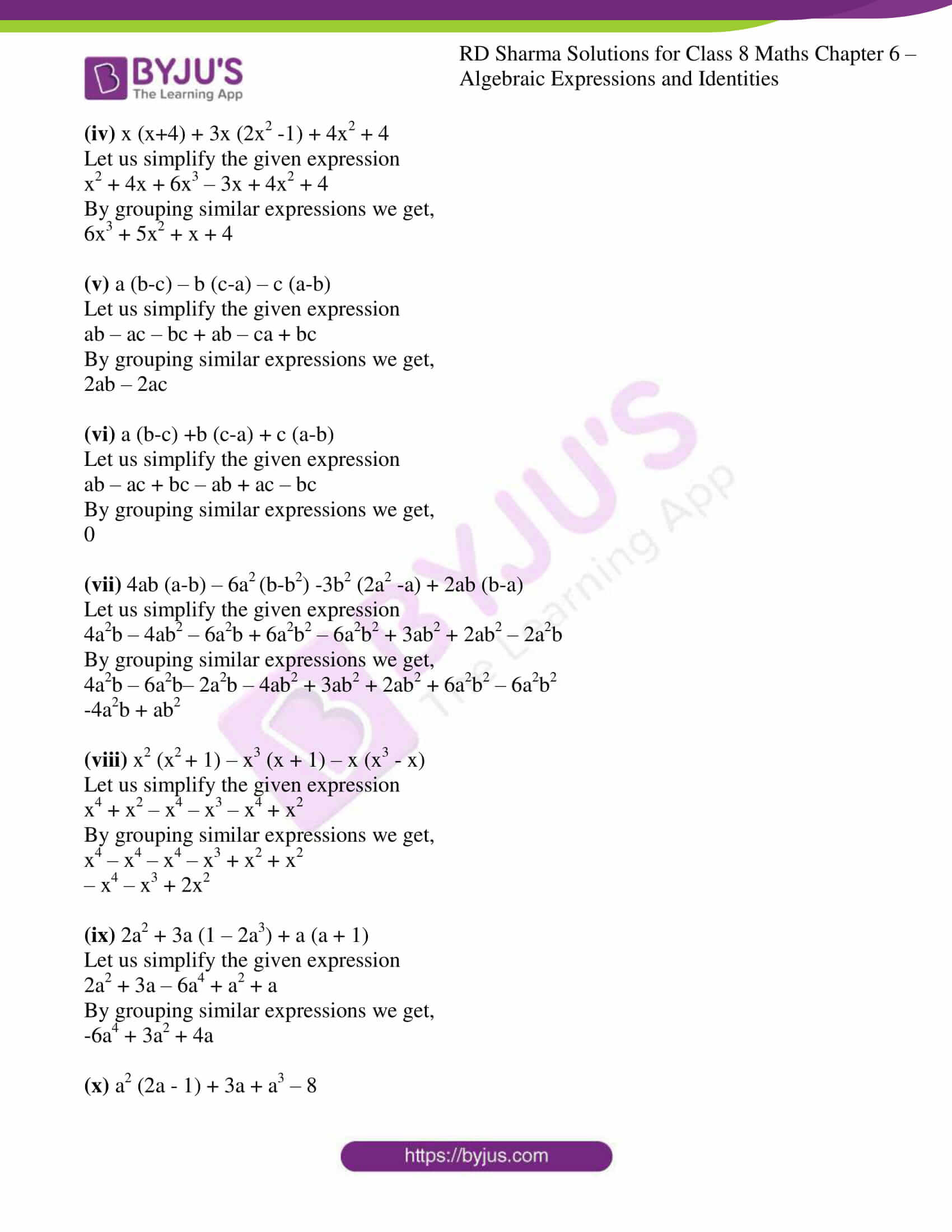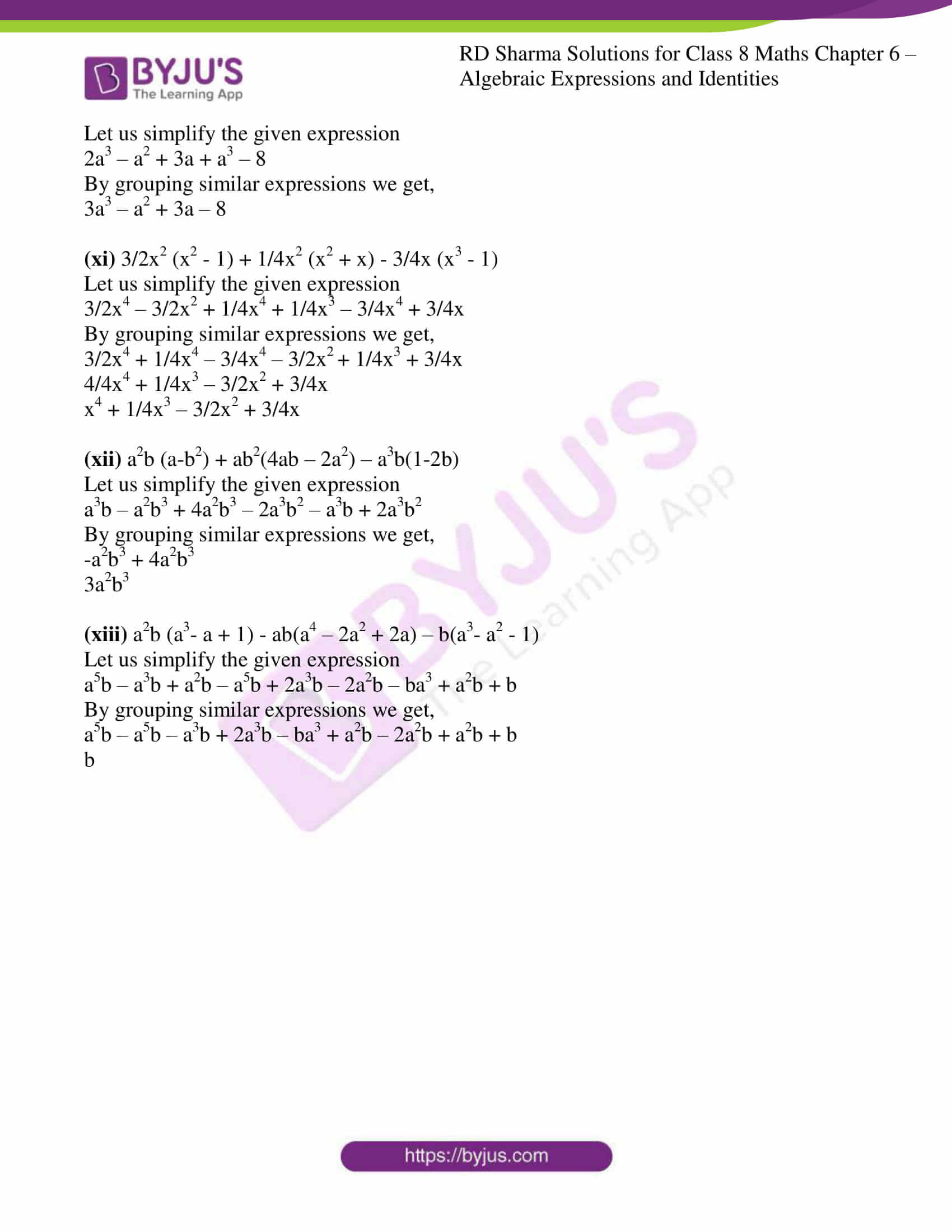### Access Answers to RD Sharma Solutions for Class 8 Maths Exercise 6.4 Chapter 6 Algebraic Expressions and Identities

#### EXERCISE 6.4 PAGE NO: 6.21

Find the following products:

1. 2a3 (3a + 5b)

Solution:

Let us simplify the given expression

2a3 (3a + 5b)

(2a3 × 3a) + (2a3 × 5b)

6a3+1 + 10a3b

6a4 + 10a3b

2. -11a (3a + 2b)

Solution:

Let us simplify the given expression

-11a (3a + 2b)

(-11a × 3a) + (-11a × 2b)

-33a2 – 22ab

3. -5a (7a – 2b)

Solution:

Let us simplify the given expression

-5a (7a – 2b)

(-5a × 7a) – (-5a × 2b)

-35a2 + 10ab

4. -11y2 (3y + 7)

Solution:

Let us simplify the given expression

-11y2 (3y + 7)

(-11y2 × 3y) + (-11y2 × 7)

-33y3 – 77y2

5. 6x/5(x3 + y3)

Solution:

Let us simplify the given expression

6/5x (x3 + y3)

(6/5x × x3) + (6/5x × y3)

6/5x4 + 6/5xy3

6. xy (x3 – y3)

Solution:

Let us simplify the given expression

xy (x3 – y3)

(xy × x3) – (xy × y3)

x4y – xy4

7. 0.1y (0.1x5 + 0.1y)

Solution:

Let us simplify the given expression

0.1y (0.1x5 + 0.1y)

(0.1y × 0.1x5) + (0.1y × 0.1y)

0.01x5y + 0.01y2

8. (-7/4ab2c – 6/25a2c2) (-50a2b2c2)

Solution:

Let us simplify the given expression

(-7/4ab2c – 6/25a2c2) (-50a2b2c2)

(-7/4ab2c × -50a2b2c2) – (6/25a2c2 × -50a2b2 × c2)

350/4a3b4c3 + 12a4b2c4

175/2a3b4c3 + 12a4b2c4

9. -8/27xyz (3/2xyz2 – 9/4xy2z3)

Solution:

Let us simplify the given expression

-8/27xyz (3/2xyz2 – 9/4xy2z3)

(-8/27xyz × 3/2xyz2) – (-8/27xyz × 9/4xy2z3)

-4/9x2y2z3 + 2/3x2y3z4

10. -4/27xyz (9/2x2yz – 3/4xyz2)

Solution:

Let us simplify the given expression

-4/27xyz (9/2x2yz – 3/4xyz2)

(-4/27xyz × 9/2x2yz) – (-4/27xyz × 3/4xyz2)

-2/3x3y2z2 + 1/9x2y2z3

11. 1.5x (10x2y – 100xy2)

Solution:

Let us simplify the given expression

1.5x (10x2y – 100xy2)

(1.5x × 10x2y) – (1.5x × 100xy2)

15x3y – 150x2y2

12. 4.1xy (1.1x – y)

Solution:

Let us simplify the given expression

4.1xy (1.1x – y)

(4.1xy × 1.1x) – (4.1xy × y)

4.51x2y – 4.1xy2

13. 250.5xy (xz + y/10)

Solution:

Let us simplify the given expression

250.5xy (xz + y/10)

(250.5xy × xz) + (250.5xy × y/10)

250.5x2yz + 25.05xy2

14. 7/5x2y (3/5xy2 + 2/5x)

Solution:

Let us simplify the given expression

7/5x2y (3/5xy2 + 2/5x)

(7/5x2y × 3/5xy2) + (7/5x2y × 2/5x)

21/25x3y3 + 14/25x3y

15. 4/3a (a2 + b2 – 3c2)

Solution:

Let us simplify the given expression

4/3a (a2 + b2 – 3c2)

(4/3a × a2) + (4/3a × b2) – (4/3a × 3c2)

4/3a3 + 4/3ab2 – 4ac2

16. Find the product 24x2 (1-2x) and evaluate its value for x = 3

Solution:

Let us simplify the given expression

24x2 (1 – 2x)

(24x2× 1) – (24x2× 2x)

24x2 – 48x3

Now let us evaluate the expression when x = 3

24x2 – 48x3

24 × (3)2 – 48 × (3)3

24 × (9) – 48 × (27)

216 – 1296

-1080

17. Find the product -3y (xy+y2) and evaluate its value for x = 4 and y = 5

Solution:

Let us simplify the given expression

-3y (xy+y2)

(-3y × xy) + (-3y × y2)

-3xy2 – 3y3

Now let us evaluate the expression when x = 4 and y = 5

-3xy2 – 3y3

-3 × (4) × (5)2 – 3 × (5)3

-300 – 375

-675

18. Multiply -3/2x2y3 by (2x-y) and verify the answer for x = 1 and y = 2

Solution:

Let us simplify the given expression

-3/2x2y3 by (2x-y)

(-3/2x2y3 × 2x) – (-3/2x2y3 × y)

-3x3y3 + 3/2x2y4

Now let us evaluate the expression when x = 1 and y = 2

-3x3y3 + 3/2x2y4

-3 × (1)4 × (2)3 + 3/2 × (1)2 × (2)4

– 3 × (8) + 3 (8)

-24+24

0

19. Multiply the monomial by the binomial and find the value of each for x = -1, y = 0.25 and z = 0.005:
(i) 15y2 (2 – 3x)
(ii) -3x (y2 + z2)
(iii) z2 (x – y)
(iv) xz (x2 + y2)

Solution:

(i) 15y2 (2 – 3x)

Let us simplify the given expression

30y2 – 45xy2

By evaluating the values in the expression x = -1, y = 25/100 and z = 5/1000

30 × (25/100)2 – 45 × (-1) × (25/100)2

30 (1/16) + 45 (1/16)

15/8 + 45/16

(30+45)/16

75/16

(ii) -3x (y2 + z2)

Let us simplify the given expression

-3xy2 + -3xz2

By evaluating the values in the expression x = -1, y = 25/100 and z = 5/1000

-3× (-1) × (25/100)2 – 3 × (-1) × (5/1000)2

(3×25×25/100×100) + (3×5×5/1000×1000)

3/16 + 3/40000

39/200

(iii) z2 (x – y)

Let us simplify the given expression

z2x – z2y

By evaluating the values in the expression x = -1, y = 25/100 and z = 5/1000

z2 (x – y)

(5/1000)2 (-1 – 25/100)

(1/40000) (-100-25/100)

(1/40000) (-125/100)

(1/40000) (-5/4)

-5/160000

-1/32000

(iv) xz (x2 + y2)

Let us simplify the given expression

x3z + xzy2

By evaluating the values in the expression x = -1, y = 25/100 and z = 5/1000

x3z + xzy2

(-1)3 × (5/1000) + (-1) × (5/1000) × (25/100)2

-1/200 – 1/16 × 1/200

-1/200 – 1/3200

By taking LCM as 3200

(-16 -1)/3200

-17/3200

20. Simplify:

(i) 2x2 (x3 – x) – 3x (x4 + 2x) – 2 (x4 – 3x2)

(ii) x3y (x2 – 2x) + 2xy (x3 – x4)

(iii) 3a2 + 2 (a+2) – 3a (2a+1)

(iv) x (x+4) + 3x (2x2 -1) + 4x2 + 4

(v) a (b-c) – b (c-a) – c (a-b)

(vi) a (b-c) +b (c-a) + c (a-b)

(vii) 4ab (a-b) – 6a2 (b-b2) -3b2 (2a2 -a) + 2ab (b-a)

(viii) x2 (x2 + 1) – x3 (x + 1) – x (x3 – x)

(ix) 2a2 + 3a (1 – 2a3) + a (a + 1)

(x) a2 (2a – 1) + 3a + a3 – 8

(xi) 3/2x2 (x2 – 1) + 1/4x2 (x2 + x) – 3/4x (x3 – 1)

(xii) a2b (a-b2) + ab2(4ab – 2a2) – a3b(1-2b)

(xiii) a2b (a3– a + 1) – ab(a4 – 2a2 + 2a) – b(a3– a2 – 1)

Solution:

(i) 2x2 (x3 – x) – 3x (x4 + 2x) – 2 (x4 – 3x2)

Let us simplify the given expression

2x5 – 2x3 – 3x5 – 6x2 – 2x4 + 6x2

By grouping similar expressions we get,

2x5 – 3x5 – 2x3 – 2x4 – 6x2 + 6x2

-x5 – 2x4 – 2x3

(ii) x3y (x2 – 2x) + 2xy (x3 – x4)

Let us simplify the given expression

x5y – 2x4y + 2x4y – 2x5y

By grouping similar expressions we get,

-x5y – 2x5y

-x5y

(iii) 3a2 + 2 (a+2) – 3a (2a+1)

Let us simplify the given expression

3a2 + 2a + 4 – 6a2 – 3a

By grouping similar expressions we get,

3a2 – 6a2 + 2a – 3a + 4

-3a2 – a + 4

(iv) x (x+4) + 3x (2x2 -1) + 4x2 + 4

Let us simplify the given expression

x2 + 4x + 6x3 – 3x + 4x2 + 4

By grouping similar expressions we get,

6x3 + 5x2 + x + 4

(v) a (b-c) – b (c-a) – c (a-b)

Let us simplify the given expression

ab – ac – bc + ab – ca + bc

By grouping similar expressions we get,

2ab – 2ac

(vi) a (b-c) +b (c-a) + c (a-b)

Let us simplify the given expression

ab – ac + bc – ab + ac – bc

By grouping similar expressions we get,

0

(vii) 4ab (a-b) – 6a2 (b-b2) -3b2 (2a2 -a) + 2ab (b-a)

Let us simplify the given expression

4a2b – 4ab2 – 6a2b + 6a2b2 – 6a2b2 + 3ab2 + 2ab2 – 2a2b

By grouping similar expressions we get,

4a2b – 6a2b– 2a2b – 4ab2 + 3ab2 + 2ab2 + 6a2b2 – 6a2b2

-4a2b + ab2

(viii) x2 (x2 + 1) – x3 (x + 1) – x (x3 – x)

Let us simplify the given expression

x4 + x2 – x4 – x3 – x4 + x2

By grouping similar expressions we get,

x4 – x4 – x4 – x3 + x2 + x2

– x4 – x3 + 2x2

(ix) 2a2 + 3a (1 – 2a3) + a (a + 1)

Let us simplify the given expression

2a2 + 3a – 6a4 + a2 + a

By grouping similar expressions we get,

-6a4 + 3a2 + 4a

(x) a2 (2a – 1) + 3a + a3 – 8

Let us simplify the given expression

2a3 – a2 + 3a + a3 – 8

By grouping similar expressions we get,

3a3 – a2 + 3a – 8

(xi) 3/2x2 (x2 – 1) + 1/4x2 (x2 + x) – 3/4x (x3 – 1)

Let us simplify the given expression

3/2x4 – 3/2x2 + 1/4x4 + 1/4x3 – 3/4x4 + 3/4x

By grouping similar expressions we get,

3/2x4 + 1/4x4 – 3/4x4 – 3/2x2 + 1/4x3 + 3/4x

4/4x4 + 1/4x3 – 3/2x2 + 3/4x

x4 + 1/4x3 – 3/2x2 + 3/4x

(xii) a2b (a-b2) + ab2(4ab – 2a2) – a3b(1-2b)

Let us simplify the given expression

a3b – a2b3 + 4a2b3 – 2a3b2 – a3b + 2a3b2

By grouping similar expressions we get,

-a2b3 + 4a2b3

3a2b3

(xiii) a2b (a3– a + 1) – ab(a4 – 2a2 + 2a) – b(a3– a2 – 1)

Let us simplify the given expression

a5b – a3b + a2b – a5b + 2a3b – 2a2b – ba3 + a2b + b

By grouping similar expressions we get,

a5b – a5b – a3b + 2a3b – ba3 + a2b – 2a2b + a2b + b

b